Name:    Bonding Practice Problems

Multiple Choice
Identify the letter of the choice that best completes the statement or answers the question.

1.

For an atom of a main group element, the number of valence electrons is equal to
 a. its number of core electrons. b. the principle quantum number of its outer shell. c. its period number. d. its group number. e. eight minus its group number.

2.

Which combination of atoms is most likely to produce a compound with ionic bonds?
 a. Al and F b. P and H c. C and O d. Si and O e. S and Br

3.

Which of the following statements are CORRECT?

 1. Ionic bonds form when one or more valence electrons are transferred from one atom to another. 2. Covalent bonds involve sharing of electrons between atoms. 3. Ionic bond formation is always exothermic; covalent bond formation is always endothermic.
 a. 1 only b. 2 only c. 3 only d. 1 and 2 e. 1, 2, and 3

4.

Which of the following compounds is expected to have the strongest ionic bonds?
 a. RbF b. NaF c. NaI d. CsBr e. CsI

5.

Which of the following molecules or ions are isoelectronic: SO2, CO2, NO2-, ClO2-?
 a. SO2 and CO2 b. SO2 and NO2- c. CO2 and ClO2- d. NO2- and ClO2- e. SO2, NO2-, and ClO2-

6.

If two or more species have the same number of electrons resulting in similar Lewis structures, they are said to be ____.
 a. neutral b. resonant structures c. symmetric d. isoelectronic e. covalent

7.

Which of the following species will have a Lewis structure most like that of the hydronium ion, H3O+?
 a. NO3- b. NH3 c. SO3 d. CO32- e. H2CO

8.

Which of the following is a correct Lewis structure for ozone, O3?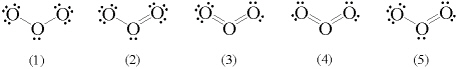a. 1 b. 2 c. 3 d. 4 e. 5

9.

Which of the following is a correct Lewis structure for the phosphate ion?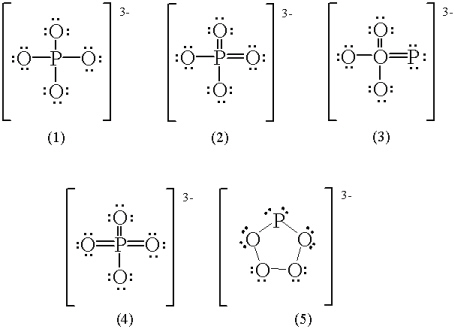a. 1 b. 2 c. 3 d. 4 e. 5

10.

Which of the following are resonance structures for the formate ion, HCO2-?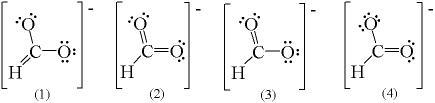a. 1 and 2 b. 2 and 3 c. 3 and 4 d. 1, 3, and 4 e. 2, 3, and 4

11.

Which of the following elements is most likely to form a molecular structure that disobeys the octet rule?
 a. B b. C c. N d. O e. F

12.

When both of the electrons in a molecular bond originate from the same atom, the bond is called a(n)
 a. double bond. b. coordinate covalent bond. c. pi bond. d. sigma bond. e. ionic bond.

13.

The central atom in XeF4 is surrounded by
 a. 3 single bonds, 1 double bond, and no lone pairs of electrons. b. 2 single bonds, 2 double bonds, and no lone pairs of electrons. c. 3 single bonds, 1 double bond, and 1 lone pair of electrons. d. 4 single bonds, no double bonds, and no lone pairs of electrons. e. 4 single bonds, no double bonds, and 2 lone pairs of electrons.

14.

Use VSEPR theory to predict the molecular geometry of H3O+.
 a. trigonal-pyramidal b. trigonal-planar c. bent d. T-shaped e. linear

15.

Use VSEPR theory to predict the molecular geometry of CS2.
 a. linear b. bent c. trigonal-planar d. tetrahedral e. octahedral

16.

Use VSEPR theory to predict the molecular geometry of IF5.
 a. tetrahedral b. see-saw c. trigonal-bipyramidal d. square-pyramidal e. octahedral

17.

Use VSEPR theory to predict the molecular geometry of CO32-.
 a. bent b. tetrahedral c. trigonal-pyramidal d. T-shaped e. trigonal-planar

18.

What are the Cl-P-Cl bond angles in PCl3?
 a. 109.5° b. 120° c. 109.5° and 120° d. 90° and 120° e. 90° and 180°

19.

What is the bond angle in N2O?
 a. 90° b. 107° c. 109.5° d. 120° e. 180°

20.

Place the following molecules in order from smallest to largest bond angles: NH4+, NH3, and NH2-.
 a. NH4+ < NH3 < NH2- b. NH4+ < NH2- < NH3 c. NH2- < NH3 < NH4+ d. NH2- < NH4+ < NH3 e. NH3 < NH2- < NH4+

21.

What is the formal charge on each atom in a hypobromite ion, OBr-?
 a. O = -2, Br = -1 b. O = -2, Br = +1 c. O = -1, Br = +1 d. O = -1, Br = 0 e. O = 0, Br = -1

22.

One resonance structure for thiocyanate ion is drawn below. What is the formal charge on each atom?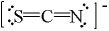a. S = 0, C = -1, N = 0 b. S = 0, C = 0, N = -1 c. S = -1, C = 0, N = 0 d. S = 0, C = +2, N = -3 e. S = -2, C = +4, N = -3

23.

Predict which of the following compounds will have the most polar covalent bond(s).
 a. HF b. CBr4 c. H2S d. NCl3 e. HI

24.

Which one of the following molecules has a dipole moment?
 a. CI4 b. PF5 c. NCl3 d. SO3 e. O2

25.

In molecules, as bond order increases,
 a. both bond length and bond energy increase. b. both bond length and bond energy decrease. c. bond length increases and bond energy is unchanged. d. bond length is unchanged and bond energy increases. e. bond length decreases and bond energy increases.

26.

How many sigma (s) bonds and pi (p) bonds are in the following molecule?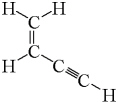a. five s and two p b. five s and three p c. five s and five p d. seven s and two p e. seven s and three p

27.

How many sigma (s) bonds and pi (p) bonds are in acetic acid?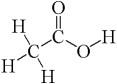a. six s and one p b. six s and two p c. seven s and one p d. eight s and zero p e. eight s and one p

28.

To form a set of sp3d2 hybrid atomic orbitals, what set of pure atomic orbitals must be mixed?
 a. one s, one p, and one d b. one s, three p, and one d c. one s, three p, and two d d. two s, six p, and two d e. two s, six p, and four d

29.

What is the maximum number of hybridized orbitals formed by an oxygen atom?
 a. 2 b. 3 c. 4 d. 5 e. 6

30.

What is the hybridization of the carbon atom in carbon disulfide?
 a. sp b. sp2 c. sp3 d. sp3d e. sp3d2

31.

What is the hybridization of the sulfur atom in SO2?
 a. sp b. sp2 c. sp3 d. sp3d e. sp3d2

32.

What is the hybridization of the sulfur atom in SF4?
 a. sp b. sp2 c. sp3 d. sp3d e. sp3d2

33.

What is the hybridization of the central atom in a molecule with a square-planar molecular geometry?
 a. sp b. sp2 c. sp3 d. sp3d e. sp3d2

34.

What is the hybridization of the central atom in a molecule with a trigonal-planar geometry?
 a. sp b. sp2 c. sp3 d. sp3d e. sp3d2

35.

In which of the following molecules and ions does the central carbon atom have sp hybridization: Cl2CO, CH2Br2, CO2, and OCN-?
 a. Cl2CO only b. Cl2CO and CH2Br2 c. CH2Br2 and CO2 d. CH2Br2 and OCN- e. CO2 and OCN-

36.

What is the molecular geometry around a central atom that is sp3 hybridized and has one lone electron-pair?
 a. bent b. linear c. trigonal-planar d. trigonal-pyramidal e. trigonal-bipyramidal

37.

What is the molecular geometry around a central atom that is sp2 hybridized, has three sigma bonds, and has one pi bond?
 a. trigonal-planar b. trigonal-pyramidal c. trigonal-bipyramidal d. linear e. tetrahedral

38.

What is the molecular geometry around a central atom that is sp3d hybridized and has three sigma bonds and two lone electron-pairs?
 a. linear b. trigonal-pyramidal c. trigonal-planar d. tetrahedral e. T-shaped

39.

What is the molecular geometry around a central atom that has four sigma bonds and has no lone electron-pairs?
 a. square-pyramidal b. tetrahedral c. trigonal-planar d. square-planar e. see-saw

40.

Which of the following characteristics apply to PCl3?

 1. nonpolar molecule 2. polar bonds 3. trigonal-pyramidal molecular geometry 4. sp2 hybridized
 a. 1 and 2 b. 2 and 3 c. 3 and 4 d. 1, 2, and 3 e. 1, 2, 3, and 4

41.

Which of the underlined atoms (C1, C2, N, and O) are sp2 hybridized?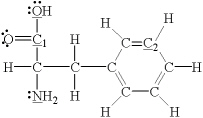a. C1 and C2 b. C1, N, and O c. N and O d. O and C2 e. O only

42.

Which of the following statements are CORRECT?

 1. A paramagnetic substance is attracted to a magnetic field. 2. Atoms with one or more unpaired electrons are paramagnetic. 3. Electron spin is quantized.
 a. 1 only b. 2 only c. 3 only d. 1 and 2 e. 1, 2, and 3

43.

Which of the following statements are CORRECT?

 1. Substances that retain their magnetism after they are withdrawn from a magnetic field are called ferromagnetic. 2. A diamagnetic substance is slightly repelled by a magnetic field. 3. An atom with an even number of electrons is diamagnetic.
 a. 1 only b. 2 only c. 3 only d. 1 and 2 e. 1, 2, and 3

44.

Which of the following atoms is paramagnetic?
 a. Se b. Cd c. Ar d. He e. Ca